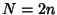## Even Number

An Integer of the form, whereis an Integer. The even numbers are therefore ...,,, 0, 2, 4, 6, 8, 10, ... (Sloane's A005843). Since the even numbers are integrally divisible by two,for even. An even numberfor whichis called a Singly Even Number, and an even numberfor whichis called a Doubly Even Number. An integer which is not even is called an Odd Number. The Generating Function of the even numbers isSee also Doubly Even Number, Even Function, Odd Number, Singly Even Number

References

Sloane, N. J. A. Sequence A005843/M0985 in An On-Line Version of the Encyclopedia of Integer Sequences.'' http://www.research.att.com/~njas/sequences/eisonline.html and Sloane, N. J. A. and Plouffe, S. The Encyclopedia of Integer Sequences. San Diego: Academic Press, 1995.